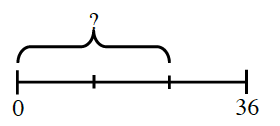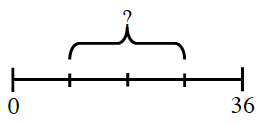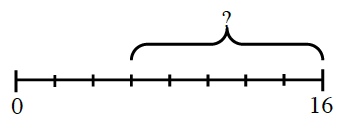### Home > CC2 > Chapter Ch4 > Lesson 4.3.3 > Problem4-120

4-120.

Identify the length of the missing section of each line. Assume that the lines are divided into equal parts. Homework Help ✎

1.How many segments is $36$ broken into? Use this to figure out the value of each segment.

2.How many segments is 36 broken into? Use this to figure out the value of each segment.

$18$. Be sure to show why this answer is correct.

3.How is this problem the same or different than parts (a) and (b)?